Next: 2.2 Vector quantization Up: 2.1 Principal component analysis Previous: 2.1.1 Neural networks for

## 2.1.2 Probabilistic PCA

Probabilistic PCA links PCA to the probability density of patterns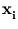(Tipping and Bishop, 1997). The given set {} is assumed to originate from a probability density p(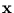). Further,is assumed to be a linear combination of a vector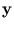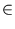IRq with density p() and a noise vector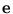IRd with density p(),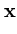=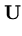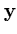+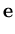. (2.7)

The goal is to find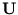, which is a d×q matrix. Both densities p() and p() are assumed to be uniformly Gaussian with variance one respective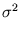. Thus, the density p() is defined uniquely up to the parametersand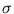,

 p() = (2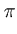)-d/2(det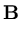)-1/2exp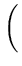-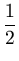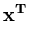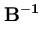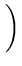(2.8)

with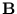=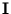+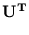(Tipping and Bishop, 1997). Probabilistic PCA determinesandsuch that the patternsif drawn from p() are most likely (Tipping and Bishop, 1997). That is, the likelihood, which is

 L =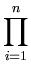p(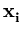) , (2.9)

is maximized (see appendix A.2 for an example of the maximum likelihood principle). The result of this optimization gives the matrix(Tipping and Bishop, 1997),=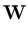(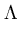-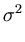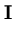)1/2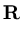. (2.10)

The columns of the matrix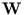are the eigenvectors of the covariance matrix of {}; the diagonal matrix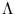contains the corresponding eigenvalues, and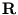is an arbitrary rotational matrix (note,has a uniform Gaussian distribution). The noise varianceturns out to be the residual variance per dimension,=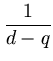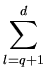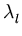. (2.11)

To evaluate (2.11), only the q principal eigenvalues and the total variance (sum of variances over all dimensions, which equals the trace of the covariance matrix) need to be known. It is not necessary to compute the d - q minor principal components. Thus, the introduction of the noise allows the density p() to be defined over the whole IRd, while using a reduced parameter set (obtained by PCA). Equation (2.11) shows how fast p() decreases orthogonal to the subspace spanned by the principal components.Next: 2.2 Vector quantization Up: 2.1 Principal component analysis Previous: 2.1.1 Neural networks for
Heiko Hoffmann
2005-03-22